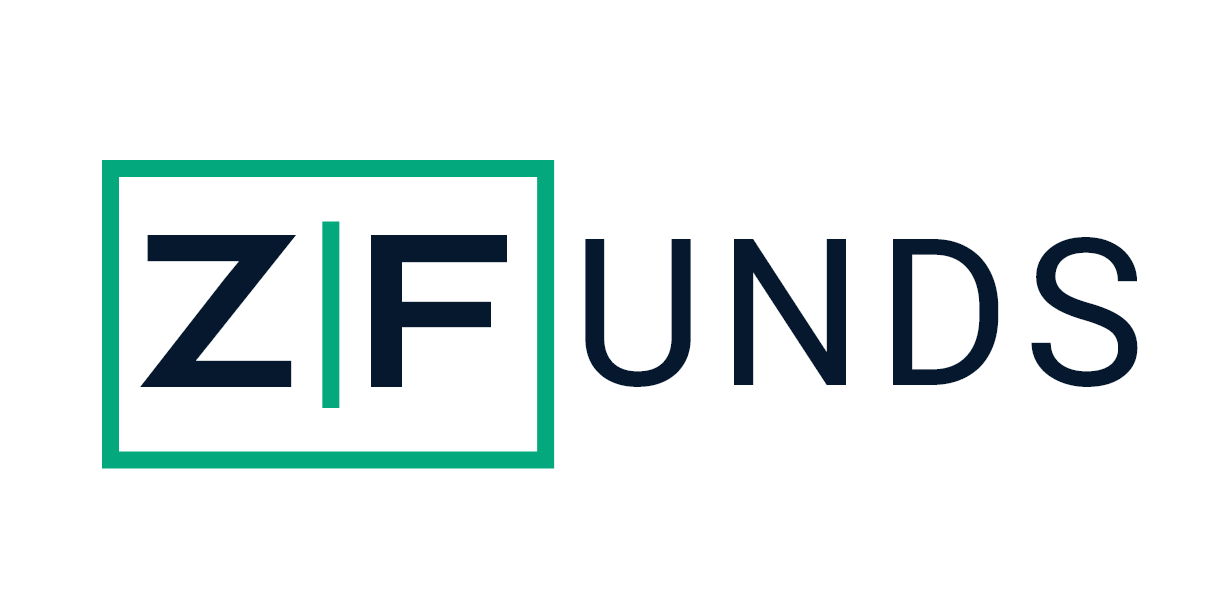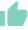# What is Sharpe Ratio - Formula, Calculation & Comparison with Information RatioGaurav Seth
20 Likes | 912 days agoLikeShareAuthor is a Zfunds Verified ExpertGaurav Seth
Gurugram## What is the Sharpe Ratio?

Nobel laureate William F. Sharpe created the Sharpe ratio in 1966 and since then it has been one of the preferred measures of return relative to the risk of an investment. The Sharpe ratio is used to assess a mutual fund's risk-adjusted performance. This ratio tells an investor the alpha or the excess return generated because of owning a risky asset.

The returns received over and above the return earned by a risk-free asset such as a fixed deposit or government bond are usually risk-adjusted returns. In terms of the "added risk" that an investor takes when investing in volatile investments such as equity funds, high returns are seen. Using the standard deviation, the risk inherent in an investment is calculated. Thus, for each additional unit of risk taken by the fund, a higher Sharpe ratio indicates a fund's better return yielding capacity.

## Uses of Sharpe Ratio -

Sharpe ratio is an accurate method to evaluate the efficiency of a fund against a unit level of risk. The higher a portfolio's Sharpe ratio, the greater its risk-adjusted-performance. If you get a negative Sharpe ratio, however, then it implies that you will be safer suited to investing in a risk-free asset than the one you are actually investing in.

2. Helps in comparing against the benchmark

Investors can compare peer funds with their existing or preferred funds. Investors may also consider the output of their current or preferred portfolios.

3. Comparison of funds measurement

The Sharpe ratio can be used as a method to compare the funds placed in the same category as a performance analysis. Through this method, you can confirm that the risk levels are comparable and so are the risk-adjusted returns. You may also compare funds that offer the same returns but are at different risk thresholds.

4. To analyze the efficiency of the Fund

The Sharpe Ratio throws light on the effectiveness of a portfolio. In comparison to the extra risks taken on in the portfolio, investors can determine the excess return created by each investment by looking at this ratio. This calculator can be used to evaluate growth style, valuation style, or a combination of all funds.

5. Analyzing the diversification of portfolios

The Sharpe Ratio can be used by investors as a technique for assessing the need for diversification of portfolios. If an investor is investing in a 2.00 Sharpe Ratio fund, it would help to reduce the ratio and risk factor by adding another fund to his portfolio.

## How to calculate the Sharpe ratio?

You will easily find the Sharpe ratio in a mutual fund's fact sheet. In case an investor prefers to calculate it himself, one needs to deduct the risk-free return from the portfolio return, which is defined as the excess return. After that, the excess return is divided by the standard deviation of the return from the portfolio. This determines the excess return for each unit of risk taken by the fund. This is known as the Sharpe ratio.

Sharpe Ratio of a fund = (Average fund returns − Risk-Free Rate) / Standard Deviation of fund returns

Example - If a fund achieves returns of 7%, while the risk-free rate is 4%, then the excess returns or the alpha generated by the fund is 3%. Let us assume the standard deviation of the funds returns are 1.5. In this case, the Sharpe ratio is 2 (3/1.5). This implies that the fund produces an additional return of 2 for every additional unit of risk taken. To maintain its Sharpe ratio at high levels, a fund with a larger standard deviation would need to produce higher returns.

## Difference Between Sharpe Ratio and Information Ratio

The Information Ratio is a measure used to calculate the risk-adjusted returns of a portfolio or security in comparison to its benchmark. The unit of risk used here is the volatility of the tracking error or the excess returns of the portfolio over its benchmark. The Sharpe Ratio, on the other hand, measures a portfolio’s risk-adjusted returns in comparison to the risk-free rate and uses the standard deviation of the portfolio returns to measure risk.

## Limitations of Sharpe Ratio

• Sharpe Ratio alone as a quantitative instrument to assess mutual fund's success is not so effective. For accurate comparison and review, it should be compared with other funds of the same group.
• Sharpe Ratio does not offer any detail on the vulnerability of the fund, whether or not the equity investment is weighted against a single field. If it is, then the risk on investment is very high, which the Sharpe ratio of the fund does not reflect
• The Sharpe Ratio formula takes the denominator's standard deviation of mutual fund returns to achieve its value. The basic premise here is that returns are invariably distributed.

• What is Sharpe Ratio?

Sharpe ratio measures the portfolio risk-adjusted returns in comparison to the risk-free rate. To measure the risk, it uses the standard deviation of the portfolio. In other words, it tells about how much excess return has been generated for each unit of risk.

• What are the uses of the Sharpe ratio?

There are many uses of the Sharpe ratio: